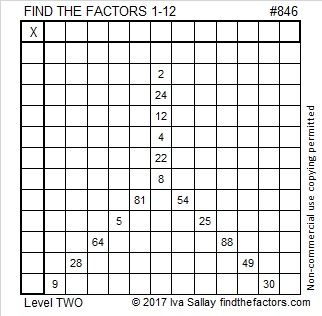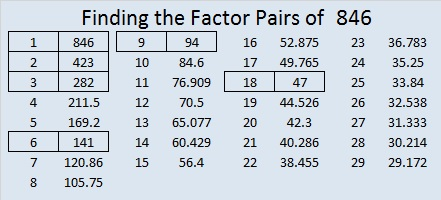# 846 and Level 2Print the puzzles or type the solution on this excel file: 12 factors 843-852

844, 845, 846, 847, and 848 are the smallest five consecutive numbers whose square roots can be simplified.

846 can be written as the sum of consecutive prime numbers two different ways. Together they use ALL the prime numbers from 13 to 127 exactly one time.

• 13 + 17 + 19 + 23 + 29 + 31 + 37 + 41 + 43 + 47 + 53 + 59 + 61 + 67 + 71 + 73 + 79 + 83 = 846; that’s eighteen consecutive primes.
• 89 + 97 + 101 + 103 + 107 + 109 + 113 + 127 = 846; that’s eight consecutive primes.

OEIS.org informs us that 846² = 715,716. Not quite a Ruth Aaron pair, but still quite impressive.

• 846 is a composite number.
• Prime factorization: 846 = 2 × 3 × 3 × 47, which can be written 846 = 2 × 3² × 47
• The exponents in the prime factorization are 2, 1, and 1. Adding one to each and multiplying we get (1 + 1)(2 + 1)(1 + 1) = 2 × 3 × 2 = 12. Therefore 846 has exactly 12 factors.
• Factors of 846: 1, 2, 3, 6, 9, 18, 47, 94, 141, 282, 423, 846
• Factor pairs: 846 = 1 × 846, 2 × 423, 3 × 282, 6 × 141, 9 × 94, or 18 × 47
• Taking the factor pair with the largest square number factor, we get √846 = (√9)(√94) = 2√209 ≈ 29.086079This site uses Akismet to reduce spam. Learn how your comment data is processed.